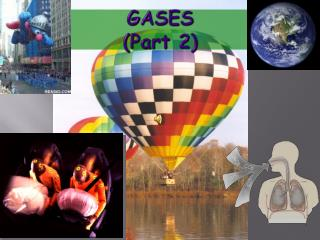DownloadDownload PresentationGASES (Part 2)

# GASES (Part 2)

Download Presentation## GASES (Part 2)

- - - - - - - - - - - - - - - - - - - - - - - - - - - E N D - - - - - - - - - - - - - - - - - - - - - - - - - - -
##### Presentation Transcript

1. GASES(Part 2)

2. twice as many molecules Avogadro’s Law Equal volumes of gases at the same T and P have the same number of molecules. V = kn V and n are directly related. MOLEY…MOLEY… MOLEY!!! V1 = V2 n1 n2

3. STP Values Standard Pressure = 1 atm Standard Temperature = 0˚ C (273 K) Standard Volume 22.4 L with 1 mole

4. Bring all the laws together PV=k V/T=k P/T=k V/n=k PV nT then =k k is given the variable R, and is called the Ideal Gas Constant So…

5. IDEAL GAS LAW P V = n R T Brings together gas properties. Can be derived from experiment and theory. BE SURE YOU KNOW THIS EQUATION!

6. Using PV = nRT P = Pressure V = Volume T = Temperature N = number of moles R is a constant, called the Ideal Gas Constant Instead of learning a different value for R for all the possible unit combinations, we can just memorizeonevalue and convert the units to match R. R = 0.08206 L • atm mol • K

7. Using PV = nRT How much N2 is required to fill a small room with a volume of 960 cubic feet (27,000 L) to 745 mm Hg at 250 C? Solution 1. Get all data into proper units V = 27,000 L T = 250 C + 273 = 298 K P = 745 mm Hg (1 atm/760 mm Hg) = 0.980 atm And we always know R, 0.08206 L atm / mol K

8. Using PV = nRT How much N2 is req’d to fill a small room with a volume of 960 cubic feet (27,000 L) to P = 745 mm Hg at 250 C? Solution 2. Now plug in those values and solve for the unknown. PV = nRT RT RT n = (0.980 atm) (27,000 L) _ (0.08206) (298 K) n = 1.1 x 10 -3 mol

9. Learning Check Dinitrogen monoxide (N2O), laughing gas, is used by dentists as an anesthetic. If 2.86 mol of gas occupies a 20.0 L tank at 23°C, what is the pressure (mm Hg) in the tank in the dentist office? P(20.0 L) = (2.86moles)(0.08206)(296K) P = 3.47 atm P = 3.47 x 760 mmHg 1 atm P = 2640 mmHg

10. Learning Check A 5.0 L cylinder contains oxygen gas at 20.0°C and 735 mm Hg. How many grams of oxygen are in the cylinder? (.97atm)(5.0L)=n(0.08206)(293K) 4.85 = 24.04n .20 moles = n .20 moles O2 x 32.00 g O2 1 mole = 6.4 grams O2